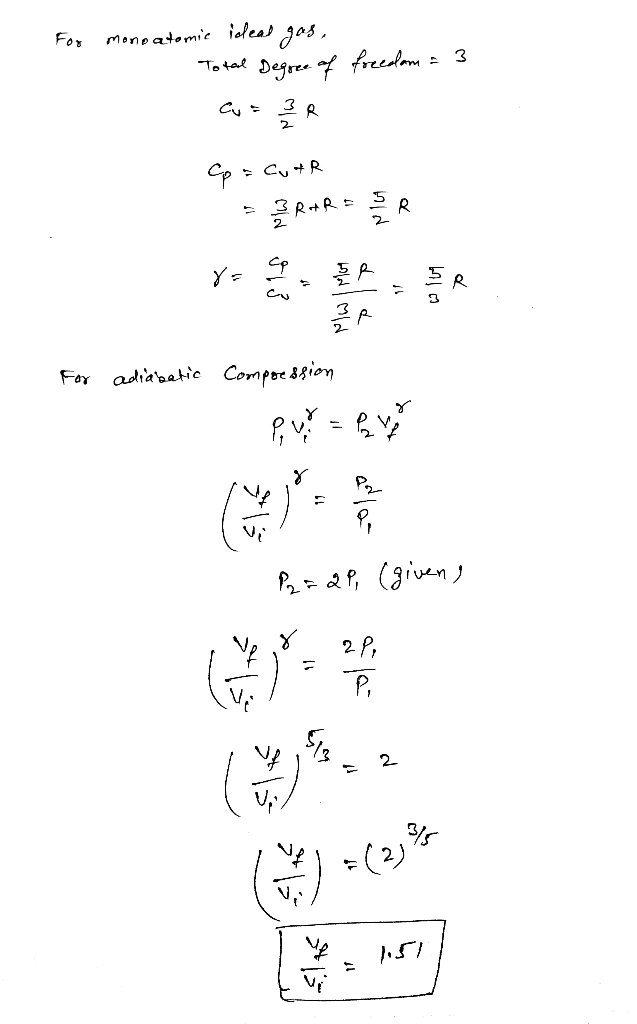In: Chemistry

# The pressure of a monatomic ideal gas doubles during an adiabatic compression. What is the ratio...

The pressure of a monatomic ideal gas doubles during an adiabatic compression. What is the ratio of the final volume to the initial volume (Vf/Vi)?

## Solutions

##### Expert Solution## Related Solutions

##### An ideal Otto cycle has a compression ratio of 8. At the beginning of the compression...
An ideal Otto cycle has a compression ratio of 8. At the beginning of the compression process, air is at 95 kPa and 27°C, and 900 kJ/kg of heat is transferred to air during the constant-volume heat-addition process. Taking into account the variation of specific heats with temperature, determine (a) the pressure and temperature at the end of the heat-addition process, (b) the net work output, (c) the thermal efficiency, and (d) the mean effective pressure for the cycle
##### An ideal Otto cycle has a compression ratio of 7. At the beginning of the compression...
An ideal Otto cycle has a compression ratio of 7. At the beginning of the compression process, air is at 98 kPa, 30oC and 766 kJ/kg of heat is transferred to air during the constant-volume heat addition process. Determine (a) the pressure (p3) and temperature (T3) at the end of the heat addition process, (b) the net work output, (c) the thermal efficiency and (d) the mean effective pressure for the cycle. Use the IG model
##### PART A A sample of ideal gas is in a sealed container. The pressure of the...
PART A A sample of ideal gas is in a sealed container. The pressure of the gas is 735 torr , and the temperature is 22 ∘C . If the temperature changes to 76 ∘C with no change in volume or amount of gas, what is the new pressure, P2, of the gas inside the container? Express your answer with the appropriate units. PART B Using the same sample of gas (P1 = 735 torr , T1 = 22 ∘C...
##### What is the ideal gas law constant?
What is the ideal gas law constant?please give details.
##### a simple ideal Brayton cycle with air as the working fluid has a pressure ratio of...
a simple ideal Brayton cycle with air as the working fluid has a pressure ratio of 10. The air enters the compressor at 520 R and the turbine at 2000 R. Accounting for the variation of specific heats with temperature, determine (a) the ait temperature at the compressor exit, (b) the back work ratio, and (c) the thermal efficiency.
##### A Diesel engine with a compression ratio of 16 has a cutoff ratio of 1.5. The...
A Diesel engine with a compression ratio of 16 has a cutoff ratio of 1.5. The state before polytropic compression (exponent 1.3) is 1700 cm3 , 96 kPa and 22°C and there is isentropic expansion. (a) Sketch the P-v diagram for this cycle. (b) Calculate the heat transfer in and heat transfer out. answer must be Qin = 657 J or 724 J or 866 J, Qout = 291 J or 296 J or (c) Determine the thermal efficiency. answer...
##### A Diesel engine with a compression ratio of 16 has a cutoff ratio of 1.5. The...
A Diesel engine with a compression ratio of 16 has a cutoff ratio of 1.5. The state before polytropic compression (exponent 1.3) is 1700 cm3, 96 kPa and 22°C and there is isentropic expansion. (a) Sketch the P-v diagram for this cycle. (b) Calculate the heat transfer in and heat transfer out. (c) Determine the thermal efficiency. (d) Calculate the mean effective pressure.
##### In the van der Waals model of a gas, as compared to an ideal gas, a....
In the van der Waals model of a gas, as compared to an ideal gas, a. Intermolecular forces decrease the pressure, but finite molecular volume increases it b. Both intermolecular forces and finite molecular volume increase the pressure c. Both intermolecular forces and finite molecular volume decrease the pressure d. Intermolecular forces increase the pressure, but finite molecular volume decreases it
##### By what factor does the pressure of the gas increase if the gas is compressed to half its original volume?
A cylinder with a movable piston contains 3 moles of hydrogen at standard temperature and pressure. The walls of the cylinder are made of a heat insulator, and the piston is insulated by having a pile of sand on it. By what factor does the pressure of the gas increase if the gas is compressed to half its original volume?
##### 1) Derive the Clausius-Clapeyron equation for an ideal gas and ideal liquid: Liquid (Phase A) ↔...
1) Derive the Clausius-Clapeyron equation for an ideal gas and ideal liquid: Liquid (Phase A) ↔ Gas (Phase B) Begin with the equation relating chemical potential of the two phases (?A , ?B ), assuming these phases are at equilibrium (as they are assumed to be along the liquid-gas coexistence curve on a P-T phase diagram) Include all necessary detail in your derivation to demonstrate your understanding. Then, Using the equation you derived in number 1, determine at which pressure...